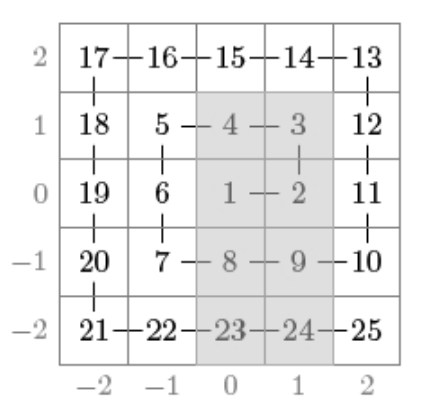시간 제한메모리 제한제출정답맞힌 사람정답 비율
1.5 초 256 MB74466.667%

## 문제

A grid of size (2n + 1) × (2n + 1) has been constructed as follows. Number 1 has been placed in the center square, number 2 has been placed to the right of it, and the following numbers have been placed along the spiral counterclockwise.

Your task is to calculate answers for q queries where the sum of numbers in an rectangular region in the grid is requested (modulo 109 + 7). For example, in the following grid n = 2 and the sum of numbers in the gray region is 74:## 입력

The first input line contains two integers n and q: the size of the grid and the number of queries.

After this, there are q lines, each containing four integers x1, y1, x2 and y2 (-n ≤ x1 ≤ x2 ≤ n, -n ≤ y1 ≤ y2 ≤ n). This means that you should calculate the sum of numbers in a rectangular region with corners (x1, y1) and (x2, y2).

## 출력

You should output the answer for each query (modulo 109 + 7).

## 제한

In all subtasks 1 ≤ q ≤ 100.

• 1 ≤ n ≤ 1000

## 서브태스크 2 (15점)

• 1 ≤ n ≤ 109
• x1 = x2 and y1 = y2

• 1 ≤ n ≤ 105

• 1 ≤ n ≤ 109
• x1 = y1 = 1

• 1 ≤ n ≤ 109

## 예제 입력 1

2 3
0 -2 1 1
-1 0 1 0
1 2 1 2


## 예제 출력 1

74
9
14


## 채점 및 기타 정보

• 예제는 채점하지 않는다.